# Circumscribed

Circumscribed meaning is, a shape is restricted within any other shape. There is no circumvent for the inside figure to pass the outer figure. When we draw a figure around another figure, in such a way, that the outer figure which is surrounding the inside figure touches all its vertices, but doesn’t cross it. The outer figure touches the maximum points of the inside figure without crossing it. These geometrical shapes could be a circle, a square, a triangle, a rectangle or a quadrilateral.

## Circumscribed Meaning

The meaning of circumscribed in Geometry is drawing a figure around another figure in such a way that the drawn figure touches the outer line or points of the inside figure without intersecting it. It should limit the inside shape within itself. Suppose, a regular hexagon is circumscribed by a circle, then the circle touches its six vertices without cutting it. Let us discuss more circumscribed shapes, such as:

• Circle
• Triangle
• Polygon
• Angle
• Rectangle

### Circumscribed Circle

The circle which passes through all the vertices of any given geometrical figure or a polygon, without crossing the figure. This is also termed as circumcircle.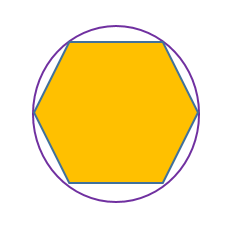In the below figure, you can see, a hexagon is inside a circle, whose all 6 vertices have been touched by the circle.

### Circumscribed Triangle

When a triangle surrounds any geometrical shape in such a way that it touches the inside figure at maximum points but never cuts it, such a triangle is called a circumscribed triangle.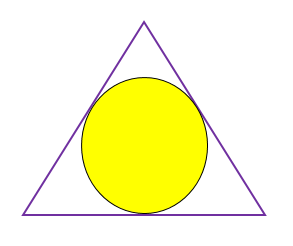We can see in the above, the triangle surrounds the circle in such a way that the sides of the triangle are tangent to the circle.

### Circumscribed Polygon

When a polygon surrounds any geometrical figure, in such a way that all the vertices inside the figure touch the sides of the polygon, then it is circumscribed. Polygon could be regular or irregular.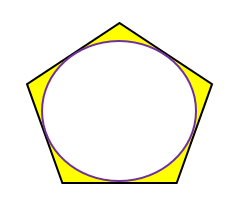In the above figure, we can see a pentagon which surrounds a circle.

### Circumscribed Angle

The circumscribed angle is the angle whose arms touch the circle to form a tangent to it.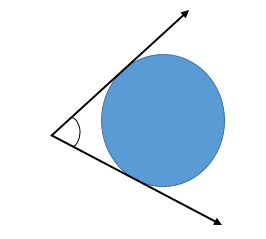In the figure above, we can see that, arms of angle are tangent to the circle. Hence, the given angle is circumscribed.

A quadrilateral which surrounds a circle, in such a way, that the sides of the quadrilateral are tangent to the circle. Then it is said to be circumscribed.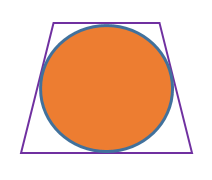We can see in the figure above quadrilateral circumscribing the circle. Here the sides of the quadrilateral are tangent to the circle. When the two pairs of sum of opposite sides are equal then the quadrilateral can circumscribe about a circle. Not all quadrilaterals are circumscribed quadrilaterals.

### Circumscribed Hexagon

A hexagon which surrounds any geometrical figure such that all the vertices of the inside figure touches the sides of the hexagon, then it is called circumscribed. When a hexagon surrounds a circle such that the sides of the hexagon are tangent to the circle we call it a circumscribed hexagon of the circle.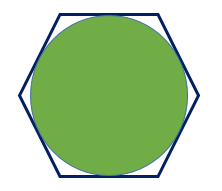In the above figure, you can see, hexagon surrounding the circle. And each side is tangent to the circle.

### Circumscribed Rectangle

A rectangle surrounding any geometrical figure touches the inside figure at its maximum points, then it is said to be circumscribed.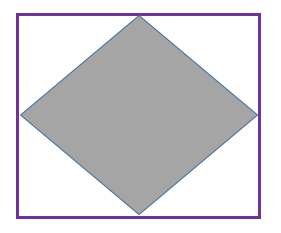In the above figure, we can see the rectangle surrounding the rhombus, touching all its 4 vertices.

## Frequently Asked Questions on Circumscribed – FAQs

Q1

### What does circumscribe mean?

When we draw a figure which touches all the vertices of another figure inside it, then we can say that the inside figure is circumscribed by the outer figure.
Q2

### What is a circumscribed triangle?

A triangle is called a circumscribed triangle when a circle passes through all three vertices of the triangle. The circumscribed circle’s centre is the triangle’s circumcenter; this is the point where the perpendicular bisectors of the sides meet.
Q3

### What is the difference between inscribed and circumscribed?

A circumscribed polygon is a polygon in which each side of the polygon is a tangent to a circle. Here, we can say that a polygon inscribed the circle. An inscribed polygon is a polygon in which all vertices lie on a circle. The polygon is inscribed in the circle, and the circle is circumscribed about the polygon.
Q4

### What shapes can be circumscribed?

Not every polygon has a circumscribed circle. Polygons that do have are called cyclic polygons, or sometimes concyclic polygons because their vertices are concyclic. All triangles, all regular simple polygons, rectangles, isosceles trapezoids, and right kites are cyclic. Hence, these shapes contain circumscribed circles.
Q5

### Which quadrilaterals have circumscribed circles?

All convex quadrilaterals which are cyclic have circumscribed circles. On the other hand, concave polygons do not have circumscribed circles.